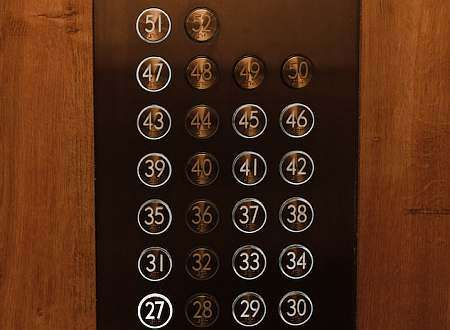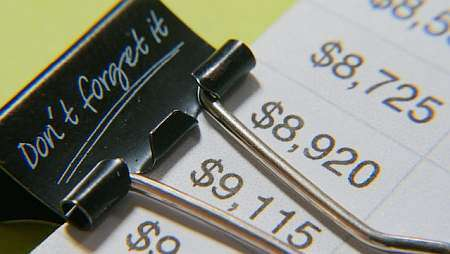# Round Calculator

Instructions: Use this round calculator to compute and round any number or numeric expression you provide, showing all the steps. Please type the number you want to round in the form box below.Enter the numeric expression you want to round (Ex: 2.344, etc.)Enter the precision for the rounding. Will be set to 0 if left empty (Ex: 2, etc)

Use this calculator to round a number you provide, showing all the steps. It does not necessarily have to be a number, you can provide a numeric expression, such as a fraction (ex: 4/5), square root (ex: sqrt(50)) or any valid numeric expression, previously simplified or not.

When you are done typing the number or numeric expression, click on "Calculate", and all the steps to compute the rounding of the number will be provided to you.

The rounding of a number is a simple process, especially when rounding to an integer (with 0 decimals of precision). When simplifying with a precision greater than 0, we need to be slightly more careful in the procedure.## How to round a number?

Rounding a number is a very common operation conducted, and it is quite easy to do, especially if a number is provided directly. If a more complicated numeric expression is provided, then you will need to numerically evaluate the number before rounding it.

Rounding will depend on the precision required. When the precision is zero (the default case), you need to round to an integer, which means, find the closest integer, that is above or below the given number.

When the precision is greater zero, you need to round to certain number of digits, this is, find the number with 2 digits that is the closest to the given number (those two digits can be zero).

## What are the steps to round a number?

• Step 1: Identify the number you want to round (and call it x), and the precision to be zero. If no precision is given, assume it is 0.
• Step 2: If the precision is 0, first determine if x is an integer, and it is is, then round(x) = x. If not, see its first decimal, if it is 5 or higher, drop all decimals, add 1 and you have found round(x), and if its first decimal is less then 5, drop all decimals to get round(x)
• Step 3: If the precision is greater than 0, say precision is k, first determine if x has at most k decimals, and if it does, then round(x) = x. If not, see its first decimal after the k-th one, and if it is 5 or higher, drop all decimals beyond the k-th one, and add 1 to the k-th decimal to get round(x), and if its first decimal after the k-th one is less than 5, drop all decimals beyond the k-th one to get round(x)

Observe that the above steps work well, except when we have number with infinite digits, and the repeated digit is '9', in which case such number can be shortened.

For example, 3.49999999999..... with infinite 9's is the same as 3.5. So then round(3.49999999999.....) = 4, but if you truncate the nines at any point, we get round(3.49999999999) = 3

## Rounding to the nearest tenth calculator

In the context of our definition, rounding to the nearest tenth calculator is the same as rounding with precision 1.

Lots of words to define a simple concept. It best see an example. To round 2.5 for example, since no precision is given, we see that 2.5 is not an integer, so we see its first decimal, which is '5', so then, round(2.5) = 3.

Now, if we want to round 2.467 with precision 2, we see that 2.467 has more than 2 decimals, so we see that the first decimal after the second is '7' which is greater than '5', so then we drop all decimals after the second and add '1', so then round(2.467) = 2.47.

## Rounding numbers to the nearest whole number

Rounding with precision equal to zero is the same as rounding to the nearest whole number. This calculator will do that by default if you don't provide any value for the precision.

Rounding numbers is perhaps easier to compute intuitively rather following the steps shown above. The thing is rounding to the nearest whole number is a very intuitive operation to conduct, but rounding to the nearest number with 2 decimal numbers could appear a bit less intuitive .

Also, there is a good reason to set a clear list of steps to follow, so you can eliminate the guess work. For example, what do you get if you round 3.4999999999? It could seem that 3.49999 is almost like 3.5, which is rounded as 4, but yet round(3.49999999) = 3, if you strictly follow the steps above.

## Is rounding numbers really necessary?

As a matter or fact, it is often times super necessary. For example, if I ask you to get the decimal expansion of 1/3, you can write something like 1/3 = 0.33333333..... This sequence does not end, so typically when expressing in paper, you need to round it.

Also, many applications have certain requirements to work with integer variables only, in which case it is very practical to be able to round those variables to get an integer value.

## How to round a number in excel

If you are working in Excel, you can use directly its "=ROUND()" function, that receives the number you want to round, and optionally you can provide the precision.

For example, to round 3.4 to the nearest integer in Excel you would use "=ROUND(3.4)", and to round 3.48 to the nearest tenth you can use "=ROUND(3.48, 1)".### Example: Rounding numbers

Round the following number: $$2.45$$

Solution: We get directly that the closest integer to 2.45 is 2, so the rounding in this case is 2.

This concludes the calculation.

### Example: Round example

Round the following expression to 2 digits: $$\frac{2}{3} + \frac{7}{4} - \frac{5}{6}$$

Solution: We need to round the given expression $$\displaystyle \frac{2}{3}+\frac{7}{4}-\frac{5}{6}$$ to the closest decimal with $$2$$ digits.

The given expression can be further reduced, and the following are the simplification steps:

$$\displaystyle \frac{2}{3}+\frac{7}{4}-\frac{5}{6}$$
$$=$$
$$\displaystyle \frac{19}{12}$$
Grouping and operating all the integer terms and fractions: $$\displaystyle \frac{ 2}{ 3}+\frac{ 7}{ 4}-\frac{ 5}{ 6}=\frac{ 2}{ 3} \times \frac{ 4}{ 4}+\frac{ 7}{ 4} \times \frac{ 3}{ 3}-\frac{ 5}{ 6} \times \frac{ 2}{ 2}=\frac{ 2 \times 4+7 \times 3-5 \times 2}{ 12}=\frac{ 8+21-10}{ 12}=\frac{ 19}{ 12}$$

Notice that in this case $$\displaystyle \frac{19}{12} \approx 1.583$$. So then, in this case, the rounding to its $$2$$-th digit is given by $$\text{round}(\frac{19}{12}) = 1.58$$.

Conclusion: The requested rounding value of the provided expression $$\displaystyle \frac{2}{3}+\frac{7}{4}-\frac{5}{6}$$ is equal to $$1.58$$.

### Example: More rounding examples

Round the following number $$-2.49999$$.

Solution: We get directly that the closest integer to -2.49999 is -2, so the rounding in this case is -2.

which concludes the calculation.

## Other Algebra calculators

Rounding provides a similar function than ceil and floor, and it is used for different purposes. Typically, we round irrational numbers that have infinite decimals, and it would be impossible to be written by extension.

Other practical calculators to have in mind are those that allow you to simplify a fraction by reducing to its lowest terms. Also, another important calculation you may be interested in is to convert fraction to percentage or also to convert a fraction to decimal.